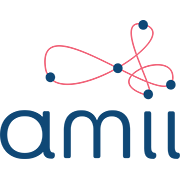課程信息

114,730 次近期查看

100% 在線

Probabilities & Expectations, basic linear algebra, basic calculus, Python 3.0 (at least 1 year), implementing algorithms from pseudocode.

您將學到的內容有

• Formalize problems as Markov Decision Processes

• Understand basic exploration methods and the exploration / exploitation tradeoff

• Understand value functions, as a general-purpose tool for optimal decision-making

• Know how to implement dynamic programming as an efficient solution approach to an industrial control problem

您將獲得的技能

• Artificial Intelligence (AI)
• Machine Learning
• Reinforcement Learning
• Function Approximation
• Intelligent Systems

100% 在線

Probabilities & Expectations, basic linear algebra, basic calculus, Python 3.0 (at least 1 year), implementing algorithms from pseudocode.

提供方阿尔伯塔大学1

Welcome to the Course!

4 個視頻 （總計 20 分鐘）, 2 個閱讀材料

An Introduction to Sequential Decision-Making

8 個視頻 （總計 46 分鐘）, 3 個閱讀材料, 2 個測驗
2

Markov Decision Processes

7 個視頻 （總計 36 分鐘）, 2 個閱讀材料, 2 個測驗
3

Value Functions & Bellman Equations

9 個視頻 （總計 56 分鐘）, 3 個閱讀材料, 2 個測驗
4

Dynamic Programming

10 個視頻 （總計 72 分鐘）, 3 個閱讀材料, 2 個測驗

關於 强化学习 專項課程